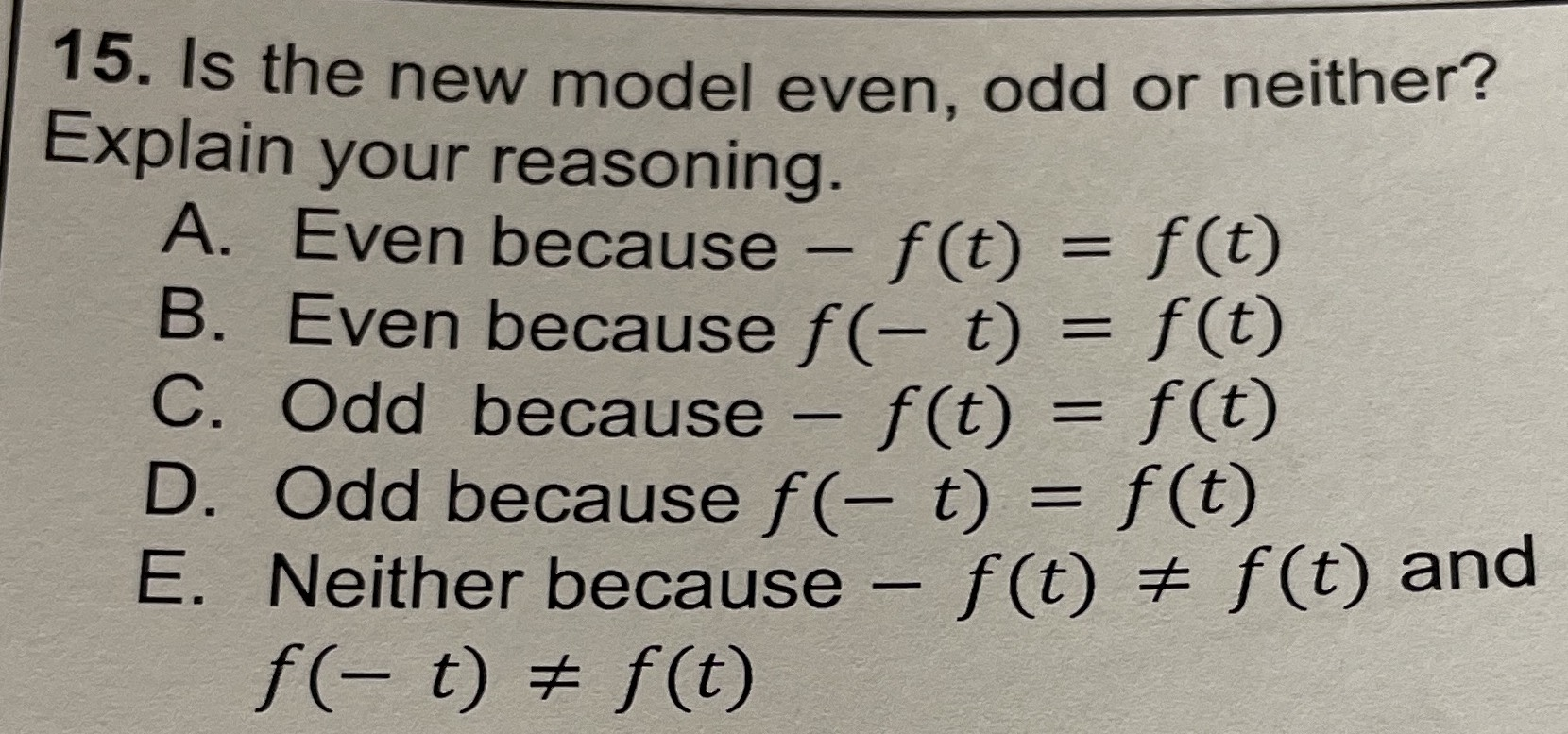### ¿Todavía tienes preguntas de matemáticas?

Pregunte a nuestros tutores expertos
Algebra
Pregunta15. Is the new model even, odd or neither? Explain your reasoning.

A. Even because $$- f ( t ) = f ( t )$$

B. Even because $$f ( - t ) = f ( t )$$

C. Odd because $$- f ( t ) = f ( t )$$

D. Odd because $$f ( - t ) = f ( t )$$

E. Neither because $$- f ( t ) \neq f ( t )$$ and $$f ( - t ) \neq f ( t )$$